Courses

# Test: Permutation And Combination- 1

## 20 Questions MCQ Test Quantitative Aptitude for GMAT | Test: Permutation And Combination- 1

Description
This mock test of Test: Permutation And Combination- 1 for GMAT helps you for every GMAT entrance exam. This contains 20 Multiple Choice Questions for GMAT Test: Permutation And Combination- 1 (mcq) to study with solutions a complete question bank. The solved questions answers in this Test: Permutation And Combination- 1 quiz give you a good mix of easy questions and tough questions. GMAT students definitely take this Test: Permutation And Combination- 1 exercise for a better result in the exam. You can find other Test: Permutation And Combination- 1 extra questions, long questions & short questions for GMAT on EduRev as well by searching above.
QUESTION: 1

### Marie has a 1 kilogram package each of two varieties of fresh apples. She also has 1 kilogram package each of three brands of canned apples. She wants to cook an apple pie using a recipe that requires one kilogram of fresh or canned apples. Assuming that she uses only one package, in how many ways can she select one package of apples for her recipe?

Solution:

Step 1: Read the question carefully & understand the objective

The objective of the question is to find the number of ways in which Marie can select ONE package of apples for her apple pie.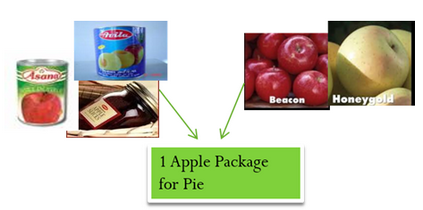The information given is:

1.  There are 2 varieties of fresh apple and 3 brands of canned apple.
2.  Quantity of apples in each fresh apple package and canned apple package is equal (1 kilogram)

Step 2:  Write the objective equation enlisting all the tasks

In order to write the objective equation, we first need to determine the tasks that need to be done to accomplish the objective.

Since Marie has to select ONE apple package for her pie, she can do the following tasks:

a. Task 1 – Select a package of fresh apples

b. Task 2 – Select a package of canned apples

Now that we know the tasks, we need to determine whether to put a multiplication or an addition sign in the objective equation.

In order to accomplish the objective, Marie can select either a fresh apple package or a canned apple package. Since doing either of the two tasks will suffice, we will put an addition sign in the objective equation.

Therefore, the objective equation will be formed as follows: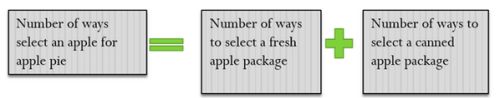Step 3: Determine the number of ways of doing each task

a. Task 1 – Select a package of fresh apples

There are two varieties of fresh apples.

This means, there are 2 ways to do Task 1.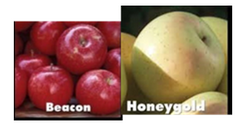b.   Task 2 – Select a package of canned apples

There are three brands of canned apples.

This means, there are 3 ways to do Task 2.Step 4: Calculate the final answer

In this step, we will plug the values in the objective equation: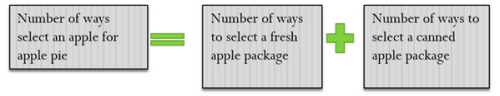= 3 + 2

= 5

So, there are 5 different ways in which Marie can select one package of apples for her recipe.

Looking at the answer choices, we see that Option C is correct.

QUESTION: 2

### There are 6 doors in a room.  In how many ways can a person enter the room through one door and exit the room through a different door?

Solution:

Step 1: Read the question carefully & understand the objective

The objective of the question is to find the number of ways in which a person can enter the room from one door and exit the room from a different door.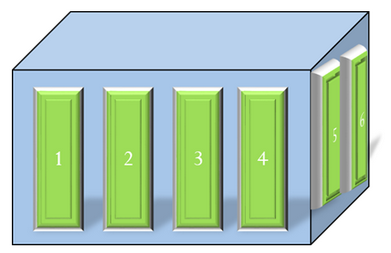The information given is:

1. There are 6 doors in the room. (Numbered as 1 to 6)
2. The person should use a different door for entry and exit. E.g. if he used Door No. 1 for entry, he should not use it for exit.

Step 2:  Write the objective equation enlisting all the tasks

In order to write the objective equation, we first need to determine the tasks that need to be done in order to accomplish the objective.

Since the person has to enter AND exit the room, the objective comprises of the following tasks:

a. Task 1 – Enter the room

b. Task 2 – Exit the room from a different door

Now, we need to determine the sign to put between the number of ways of doing each task. Since the objective statement contains the word AND between the two tasks, we will put a multiplication sign in the objective equation.

Thus, our objective equation will be: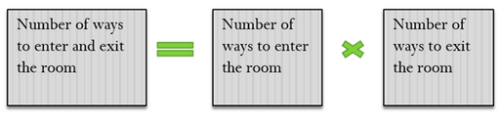Step 3: Determine the number of ways of doing each task

a. Task 1 -Enter the room

Per the information given in the question, there are 6 doors to choose to enter the room.

Thus, there are 6 ways to do Task 1.

b. Task 2 – Exit the room from a different door

Once a person has entered the room, he/she has already used one door.

Since different doors have to be used for entry and exit, i.e. repetition is not allowed, only 5 doors are left to exit the door.

So, there are 5 ways to do Task 2.

Step 4: Calculate the final answer

Now we can plug the values in the objective equation: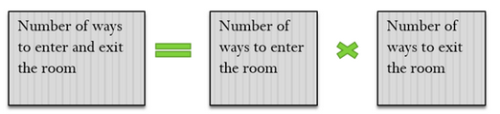=6 X 5

= 30

So, there are 30 number of ways in which a person can enter and exit a 6-door room using different doors.

Looking at the answer choices, we see that Option D is the correct answer

QUESTION: 3

### Tom lives in Dunlap and works in Peoria.  There are 5 possible routes that he can take from his home to work.  His best friend lives in Tempe and there are 8 possible routes that he can take from his home to his friend's home.  Dunlap is between Tempe and Peoria.  If he stays at his friend's place for the night, how many possible routes can he take to reach work, assuming he has to go back to his home to get ready before he goes to work? Assume that each route between Tempe and Dunlap and between Dunlap and Peoria is two-way, that is, it allows traffic from both directions.

Solution:

Step 1: Read the question carefully & understand the objective

The objective of the question is to find the number of routes to go from Tempe (Friend’s home) to Peoria (Work) via Dunlap (Tom’s home). That is, the objective of the question is to go from Tempe to Dunlap AND to go from Dunlap to Peoria.

The information given is:

1. There are 8 routes from Tempe to Dunlap
2. There are 5 routes from Dunlap to Peoria
3. Per the question, Dunlap lies somewhere in between Tempe and Peoria

Based on the above information, we can draw the following diagram: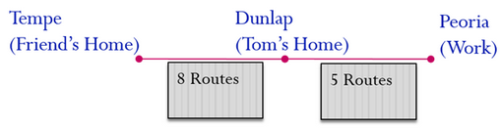Step 2:  Write the objective equation enlisting all the tasks

In order to write the objective equation, we first need to determine the tasks that need to be done to accomplish the objective.

The objective here comprises of following tasks:

a.   Task 1 – Go from Tempe (Friend’s Home) to Dunlap (Tom’s Home)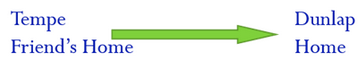b.   Task 2 – Go from Dunlap (Tom’s Home) to Peoria (Work)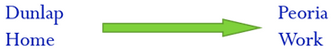Now, let’s look at the objective statement again:

“The objective of the question is to go from Tempe to Dunlap AND to go from Dunlap to Peoria.”

Since the objective equation has an AND between the two tasks, we will put a multiplication sign between the number of ways of doing each task.

Therefore, the objective equation will be: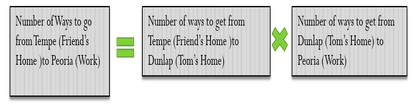Step 3: Determine the number of ways of doing each task

a. Task 1 -Go from Tempe (Friend’s Home) to Dunlap (Tom’s Home)

Per the information given in the question, there are 8 routes between Tempe and Dunlap.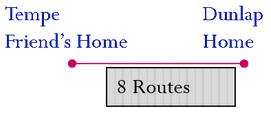Thus, there are 8 ways to do Task 1.

2.   Task 2 -Go from Dunlap (Tom’s Home) to Peoria (Work)

Per the information given in the question, there are 5 routes from Dunlap to Peoria.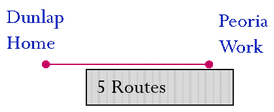Thus, there are 5 ways to do Task 2.

Step 4: Calculate the final answer

In this step, we plug the values obtained in Step 3 in the objective equation: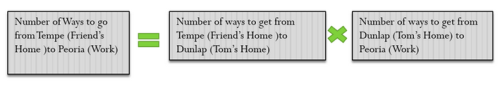= 8  X 5

= 40

So, there are 40 different routes by which Tom can go from Tempe (Friend’s Home) to Peoria (Work).

Looking at the answer choices, we see that Option E is the correct answer.

QUESTION: 4

What is the total number of outcomes possible if 6 different coins are tossed simultaneously?

Solution:

Step 1: Read the question carefully & understand the objective

As we have learnt the first step of solving any permutation and combination question is to understand the objective of the question. So, let’s do that!

The objective of the question is to find out the total number of different outcomes possible if 6 different coins are tossed at the same time.

The adjective ‘different’ used before the word ‘coins’ is of crucial importance here. Since all coins are different, this means that getting Heads on the 1st coin and Tails on all the others is a different outcome from getting Heads on the 2nd coin and Tails on all the others. (Note: there are many ways in which the 6 coins may be different. They may each be of a different denomination, or they may each of different size or color etc.)

Step 2:  Write the objective equation enlisting all the tasks

In order to write the objective equation, we first need to determine all the tasks that need to be done to accomplish the objective.

Since the person has to toss 6 coins at the same time, the objective comprises of six tasks, as listed below:

1. Task 1 – Toss the first coin
2. Task 2 – Toss the second coin
3. Task 3 – Toss the third coin
4. Task 4 – Toss the fourth coin
5. Task 5 – Toss the fifth coin
6. Task 6 – Toss the sixth coin

Now, in order to accomplish the objective, all the six tosses need to be performed. So, we will put a multiplication sign between the number of ways of doing each task.

Therefore, the objective equation will be written as under: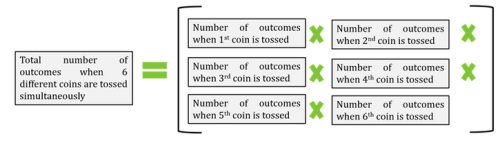Step 3: Determine the number of ways of doing each task

1. Toss the 1st coin - 2 options (Head or Tail)
2. Toss the 2nd coin - 2 options (Head or Tail)
3. Toss the 3rd coin - 2 options (Head or Tail)
4. Toss the 4th coin - 2 options (Head or Tail)
5. Toss the 5th coin - 2 options (Head or Tail)
6. Toss the 6th coin - 2 options (Head or Tail)

Step 4: Calculate the final answer

Now we can plug the values in the objective equation. We get:

2*2*2*2*2*2 = 64

So, 64 different outcomes are possible if 6 different coins are tossed simultaneously.

QUESTION: 5

One dice is picked out of two unique dices and rolled. In how many ways can a person get either a prime number on the first dice or a composite number on the second dice?

Solution:

Approach

Step 1: Read the question carefully & understand the objective

The objective of the question is to find the number of ways in which a person can get a prime number on the first dice OR a composite number on second dice.

There is not much information provided to us in the question itself. However, we can deduce a few things:

1. One of the two dices is rolled.
2. If a die is rolled there are three possible outcomes in the form of prime numbers i.e. 2, 3, and 5.
3. Similarly, there are 2 possible outcomes (4 and 6) in the form of composite numbers.

So, let’s move on to the next step in which we’ll write the objective equation.

Step 2:  Write the objective equation enlisting all the tasks

Achievement of the objective involves two tasks:

Task 1: Getting a prime number on the first dice

Task 2: Getting a composite number on the second dice

Since the objective statement contains the word 'OR' between the two tasks, we will put an addition sign between the two tasks. The objective equation will therefore be:

(Number of ways to achieve the objective) = (Number of ways to get a prime number on the first dice) + (Number of ways to get a composite number on the second dice)

Step 3: Determine the number of ways of doing each task

Now that we have the objective equation, let’s move on to the next step to find the number of ways in which these tasks can be completed.

• Task 1 - Getting a prime number on the first dice

There are three prime numbers in the numbers from 1 to 6.

Thus, there are 3 ways to do Task 1.

• Task 2 -Getting a composite number on the second dice

There are total 2 composite numbers in the range 1-6.

So, there are 2 ways to do Task 4.

Step 4: Calculate the final answer

(Number of ways to achieve the objective) = 3 + 2 = 5

So, there are 5 different ways in which a person can get a prime number on the first dice or a composite number on the second dice.

QUESTION: 6

1 boy and 6 girls are arranged in a row with 7 chairs marked A-G. How many seating arrangements are possible in which the boy sits on chair marked A?

Solution:

Since order matters in this question (Seats B, C and D occupied with Jill, Greta and Pauline is a different seating arrangement from Seats B, C and D occupied with Greta, Pauline and Jill), we can solve it using either the Filling Spaces method or the Permutation formula.

Step 1: Understand the objective

There are 7 people in this question – 1 boy and 6 girls.

And, there are 7 chairs.

Each chair is unique, because it is marked with a different number.

Chair A is reserved for the only boy in the group. So, after seating the boy on chair A, we are left with 6 chairs (marked B-G) on which we have to seat 6 girls.

The question here wants us to find the total number of seating arrangements in which the boy sits on the chair marked A. This number will be equal to the number of ways in which the 6 girls can be arranged on the chairs marked B-G.

Thus, the objective of the question here is to find the number of ways in which 6 girls can be seated on 6 seats.

Step 2: Write the objective equation enlisting all tasks

The objective here consists of a single task: Arrange 6 girls on 6 seats

Thus, the objective equation will be: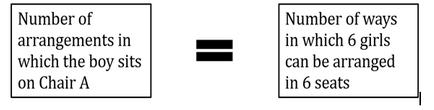Now, we know that

The number of ways in which 6 girls can be arranged in 6 seats = 6P6

So, the objective equation becomes:

(Number of arrangements in which the boy sits on Chair A) = 6P6

Step 3: Determine the number of ways of doing each task

In Step 3, using the Permutation Formula (nPn = n!), we get that

6P6 = 6! = 6*5*4*3*2*1 = 720

Step 4: Calculate the final answer

By putting these values in the objective equation, we get:

(Number of arrangements in which the boy sits on Chair A) = 720

Looking at the answer choices, we see that Option B is correct.

QUESTION: 7

How many five digit numbers can be formed with the digits 1, 2, 3, 4 and 5 if no digit can be repeated?

Solution:

Since order matters in this question (12345 is a different number from 42531), we can solve it using either the Filling Spaces method or the Permutation formula.

Step 1: Understand the objective

The objective of the question here is to find the number of 5-digit numbers that can be formed using the digits 1, 2, 3, 4 and 5 without repeating any digit.

Step 2: Write the objective equation enlisting all tasks

We will solve this question using the Permutation formula.

So, in this step, we will define the single task required to achieve the objective as: arranging 5 digits in 5 spaces

So, the objective equation can be written as:

(Number of 5 digit numbers that can be formed with the digits 1, 2, 3, 4 and 5)

= (Number of ways of arranging 5 digits in 5 spaces) = 5P5

Step 3: Determine the number of ways of doing each task

In Step 3, using the Permutation Formula (nPn = n!), we get that

5P5 = 5!

Now,

5! = 5*4*3*2*1 = 120

So, 5P5 = 120

Step 4: Calculate the final answer

By putting the value of 5P5 in the objective equation, we get:

(Number of 5 digit numbers that can be formed with the digits 1, 2, 3, 4 and 5) = 120

Looking at the answer choices, we see that Option D is correct.

QUESTION: 8

1 boy and 6 girls are arranged in a row with 7 chairs marked A-G. How many seating arrangements are possible in which the boy sits on a corner chair?

Solution:

Since order matters in this question (Seats B, C and D occupied with Jill, Greta and Pauline is a different seating arrangement from Seats B, C and D occupied with Greta, Pauline and Jill), we can solve it using either the Filling Spaces method or the Permutation formula.

Step 1: Understand the objective

There are 7 people in this question – 1 boy and 6 girls.

And, there are 7 chairs.

Each chair is unique, because it is marked with a different number.

In this question, two cases are possible:

Case 1: The boy sits on Chair A and the 6 girls are arranged on chairs B-G

Case 2: The boy sits on Chair G and the 6 girls are arranged on chairs A-F

The question here wants us to find the total number of seating arrangements in which the boy sits either on the chair A or on chair G. That is, the question wants us to find the total number of ways in which the 6 girls can be arranged either on chairs B-G or on chairs A-F. This is the objective of the question.

Step 2: Write the objective equation enlisting all tasks

The objective here consists of two tasks:

1. Task 1 – Arrange the 6 girls on the 6 seats marked B-G
2. Task 2 – Arrange the 6 girls on the 6 seats marked A-F

Next, we need to determine what sign to put in the objective equation – multiplication or addition.

Let’s look at the objective statement again:

“. . . to find the total number of ways in which the 6 girls can be arranged either on chairs B-G or on chairs A-F”

The objective statement contains the words ‘Either. . . Or’

This means that we will put an addition sign between the number of ways of doing the two tasks.

Thus, the objective equation will be: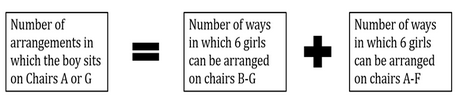Now, we know that

The number of ways in which 6 girls can be arranged in 6 seats = 6P6

So, the objective equation becomes:

(Number of arrangements in which the boy sits on chirs A or G) = 6P+ 6P6

That is,

(Number of arrangements in which the boy sits on chirs A or G) = 2 (6P6)

Step 3: Determine the number of ways of doing each task

In Step 3, using the Permutation Formula (nPn = n!), we get that

6P6 = 6! = 6*5*4*3*2*1 = 720

Step 4: Calculate the final answer

By putting these values in the objective equation, we get:

(Number of arrangements in which the boy sits on chirs A or G) = 2 x720 = 1440

Looking at the answer choices, we see that Option C is correct.

QUESTION: 9

How many five digit numbers can be formed with the digits 0, 1, 2, 3 and 4 if no digit can be repeated?

Solution:

Since order matters in this question (12340 is a different number from 42310), we can solve it using either the Filling Spaces method or the Permutation formula.

Step 1: Understand the objective

The objective of the question here is to find the number of 5-digit numbers that can be formed using the digits 0, 1, 2, 3 and 4 without repeating any digit.

Step 2: Write the objective equation enlisting all tasks

One thing to note in this question is that one of the digits is 0. When the 5 digits are arranged such that the first digit is 0 (for example: 01234), the result is a 4-digit number. The question specifically asks about the number of five digit numbers only. This means that after considering all the possible permutations of the given 5 digits, we need to subtract the cases in which the first digit is 0 (which makes the number effectively a 4-digit number)

Now, in the numbers where the first digit is 0, there are 4 digits left (1, 2, 3 and 4) to be arranged in 4 spaces.

So,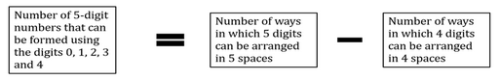This is the objective equation.

Now, we know that

The number of ways in which 5 digits can be arranged in 5 spaces = 5P5

And

The number of ways in which 4 digits can be arranged in 4 spaces = 4P4

So, the objective equation becomes:

(Number of 5-digit numbers that can be formed using the digits 0, 1, 2, 3 and 4)

= 5P5 -4P4

Step 3: Determine the number of ways of doing each task

In Step 3, using the Permutation Formula (nPn = n!), we get that

5P5 = 5! = 5*4*3*2*1 = 120

And,

4P4 = 4! = 4*3*2*1 = 24

Step 4: Calculate the final answer

By putting these valuesin the objective equation, we get:

(Number of 5-digit numbers that can be formed using the digits 0, 1, 2, 3 and 4)

= 120 – 24 = 96

Looking at the answer choices, we see that Option C is correct.

QUESTION: 10

An event is attended by 8 guests – 5 men and 3 women. 8 chairs are accordingly arranged for the guests and it is decided that the men will be seated on the chairs marked A-E and the women will be seated on the chairs marked F-H. In how many ways can the 8 guests be seated?

Solution:

Since order matters in this question (Seats A, B and C occupied with John, Graham and Paul is a different seating arrangement from Seats A, B and C occupied with Graham, Paul and John), we can solve it using either the Filling Spaces method or the Permutation formula.

Step 1: Understand the objective

The objective of the question here is to find the number of ways in which the 8 guests can be seated on 8 seats. Since the seats for men and women are fixed (Seats A-E are the 5 seats reserved for men and Seats F-H are the three seats reserved for women), the objective actually becomes: to find the number of ways in which 5 men can be arranged on 5 seats and 3 women can be arranged on 3 seats.

Step 2: Write the objective equation enlisting all tasks

The objective here consists of two tasks:

1. Task 1-Arrange 5 men on 5 seats (Seats marked A-E)
2. Task 2 – Arrange 3 women on 3 seats (Seats marked F-H)

Now that we have enlisted the tasks, we need to determine whether the number of ways of doing these two tasks should be added or multiplied.

Let’s look at the objective statement:

“To find the number of ways in which 5 men can be arranged on 5 seats and 3 women can be arranged on 3 seats.”

The objective statement contains the word AND between the two tasks. This means that we put a multiplication sign in the objective equation.

Thus, the objective equation will be: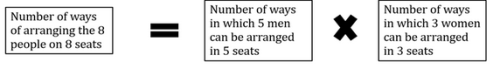Now, we know that

The number of ways in which 5 men can be arranged in 5 seats = 5P5

And

The number of ways in which 3 women can be arranged in 3 seats = 3P3

So, the objective equation becomes:

(Number of ways of arranging the 8 people on the 8 seats) = 5P5 x 3P3

Step 3: Determine the number of ways of doing each task

In Step 3, using the Permutation Formula (nPn = n!), we get that

5P5 = 5! = 5*4*3*2*1 = 120

And,

3P3 = 3! = 3*2*1 = 6

Step 4: Calculate the final answer

By putting these valuesin the objective equation, we get:

(Number of ways of arranging the 8 people on the 8 seats) = 120 x 6 = 720

Looking at the answer choices, we see that Option D is correct.

QUESTION: 11

Leslie has been given the task to write either 2-letter words or 2 digit numbers. How many unique numbers or words are possible if repetition of alphabets or digits are not allowed?

Solution:

Step 1: Understand the objective

The objective of the question is to list down unique two-digit integers or two-letter words.

The information that can be deduced from the given question is:

• Leslie can use either the digits from 0 to 9 or the alphabets A to Z to complete this activity.
• Repetition of digits or alphabets is not allowed.

Step 2: Write the objective equation enlisting all tasks

Since Leslie has to make a two-digit numbers or a two-letter words, the objective comprises of the following:

• Task 1 – Form two-digit numbers using different digits
• Task 2 – Form two-letter words using different alphabets

Now, in order to accomplish the objective, Leslie can write use either 2-digits OR 2-alphabets.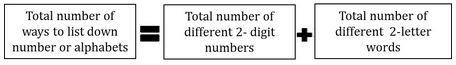Step 3: Determine the number of ways of doing each task

• Task 1 -Form two-digits numbers using digits 0 to 9.
• The number will have two different digits: one digit at the tens place and one digit at the units place.
• Now, all the 10 digits from 0 to 9 are available to us to form the 2-digit numbers.
• However, for the tens digit, we cannot choose 0 (Because a number like 09 or 02 is not a 2-digit integer, but a single digit integer)
• Therefore, for the tens digit, we can only choose from one of the digits between 1 and 9, inclusive.
• Thus, the number of ways in which the tens digit can be chosen = 9
• After one digit has been used up at the tens place, only 9 digits remain available (including 0) for the units place.
• Therefore, the number of ways in which the units digit can be chosen=9
• In order to form the two-digit numbers, we need to fill both the tens digit AND the units digit. Therefore, we will put a multiplication sign between the number of ways of filling these two places.
• Thus, Number of 2-digit numbers possible = 9*9 = 81
• Thus, there are 81 ways to do Task 1.

• Task 2 – Form two-letter words using alphabets A to Z.

• Here we have all the letters from A to Z at our disposal.
• Thus, the first place can be filled in 26 ways (anything from A to Z)
• Now that we have used one of the letters, we have 25 alphabets left.
• Since repetition of alphabets are not allowed.
• Therefore, the second place can be filled in 25 ways.
• Now, in order to form the two-letter words, we need to fill both the first place AND the second place. Therefore, we will put a multiplication sign between the number of ways of filling these two places.
• Thus, Number of 2-letter words possible = 26*25 = 650
• Thus, there are 650 ways to do Task 2

Step 4: Calculate the final answer

Now we can plug the values in the objective equation:

• Total number of different numbers or words which can be written by Leslie = 650 + 81 = 731
• So, there are 731 number of different two-digit numbers or two-letter words that can be formed if repetition is not allowed.

QUESTION: 12

There are 5 students in an English class.  The English instructor wants to hold a debate in the class in all possible groups of 2 students such that 1 student presents the case “FOR” and the other student presents the case “AGAINST”.  Each student can debate with the other student only once.  How many topics will the teacher need such that possible each pair gets a different topic?

Solution:

Step 1: Understand the objective

The objective of the question is to find the number of different debate topics the teacher will need.

And the information provided to us is:

1. There are 5 students in the class.
2. Each student debates with every other student only once.

Step 2: Write the objective equation enlisting all tasks

We need to complete the following task to accomplish the objective:

Task 1 – Make groups of 2 students by selecting 2 students from 5 students.

Thus, the Objective Equation will be:

(Number of different debate topics needed) = (Number of ways of selecting 2 students from 5 students)

Step 3: Determine the number of ways of doing each task

The number of ways in which the task can be performed = 5C2 = 10

Step 4: Calculate the final answer

Thus the teacher can make 10 groups of 2 students and hence will need to get 10 different topics.

QUESTION: 13

Stephanie wants to make a password for her home security system.  The technician has told her that she needs to make a 4-digit password and she can use all digits except 0, with no repetition.  How many passwords are possible?

Solution:

Step 1: Understand the Objective

Objective:  To find the number of 4-digit passwords.

Information:

9 digits are available, with no repetition, to form the passwords

Step 2: Write the objective equation enlisting all tasks

This is a classical PERMUTATION problem.

Task 1 – Select & Arrange 4 digits from 9 available digits to form passwords.

Number of ways to Select & Arrange = Number of 4-digit passwords

Step 3: Determine the Number of Ways for Tasks

Since this is a PERMUTATION question, permutation formula applies: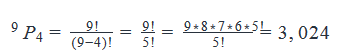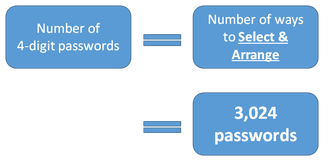Number of 4-digit passwords = 3024

QUESTION: 14

In how many ways can a room be illuminated if there are 7 bulbs in the room? Note that each bulb has different switches and the room is illuminated even if only bulb is switched on.

Solution:

Step 1: Understand the Objective

The objective of the question is to find the number of ways in which a room can be illuminated.

The information given is:

1. There are 7 bulbs in the room.
2. Each of the bulbs has a different switch.
3. Even if one bulb is switched on, the room is illuminated.

Step 2: Write the objective equation enlisting all tasks

Since it is told that the room is illuminated even if at least one bulb is switched on, we need to fulfil the following tasks to accomplish the objective:

1. Task 1 – Switch on any one bulb out of the 7
2. Task 2 – Switch on any 2 bulbs out of the 7
3. Task 3 – Switch on any 3 bulbs out of the 7
4. Task 4 – Switch on any 4 bulbs out of the 7
5. Task 5 – Switch on any 5 bulbs out of the 7
6. Task 6 – Switch on any 6 bulbs out of the 7
7. Task 7 – Switch on all the 7 bulbs

Now, in order to accomplish the objective a person can complete any of the above mentioned tasks. And hence he needs perform only of these actions. So, principle of addition will be used to form objective equation as follows: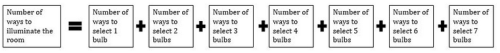Step 3: Determine the number of ways of doing each task

In this step, we’ll find out how many ways are there to perform various steps:

• Task 1 -Number of ways in which one bulb is switched on out of 7

Number of ways of selecting one items from 7 different items = 7C1 = 7

Thus, there are 7 ways to do Task 1.

• Task 2 -Number of ways in which two bulbs are switched on out of 7

Number of ways of selecting two items from 7 different items = 7C2 = 21

Thus, there are 21 ways to do Task 2.

• Task 3 -Number of ways in which three bulbs are switched on out of 7

Number of ways of selecting three items from 7 different items = 7C3 = 35

Thus, there are 35 ways to do Task 3.

• Task 4 -Number of ways in which 4 bulbs are switched on out of 7

Number of ways of selecting 4 items from 7 different items = 7C4 = 35

Thus, there are 35 ways to do Task 4.

• Task 5 -Number of ways in which 5 bulbs are switched on out of 7

Number of ways of selecting 5 items from 7 different items = 7C5 = 21

Thus, there are 21 ways to do Task 5.

• Task 6 -Number of ways in which 6 bulbs are switched on out of 7

Number of ways of selecting 6 items from 7 different items = 7C6 = 7

Thus, there are 7 ways to do Task 6.

• Task 7 -Number of ways in which 7 bulbs are switched on out of 7

Number of ways of selecting 7 items from 7 different items = 7C7 = 1

Thus, there is only one way to do Task 7.

Step 4: Calculate the final answer

(Number of ways to illuminate the room) = 7 + 21 + 35 + 35 + 21 + 7 +1

= 127

So, there are 127 different ways in which the room can be illuminated.

QUESTION: 15

Amy and Frank had a pizza party on Friday night and they had some leftovers.  They decide to eat those leftovers for lunch on Saturday.  There are 4 pizza slices all with different toppings and 2 bottles of drinks – 1 Coke and 1 Fanta.  In how many different ways can Amy and Frank eat the leftovers if they eat one pizza slice and 1 drink each?

Solution:

Step 1: Understand the Objective

The objective of the question is to find the number of ways in which Amy and Frank can have the leftovers from the pizza party.

The information given is:

1. There are 4 pizza slices with different toppings.
2. There is 1 Coke and 1 Fanta left in the drinks.
3. Each one of Frank and Amy is going to have 1 pizza slice and 1 drink.

Step 2: Write the objective equation enlisting all tasks

Let’s determine the tasks that need to be completed to accomplish the objective:

Since Frank and Amy want to have one pizza slice and one drink each, they need to fulfil the following tasks:

1. Task 1 – Select one of the four different pizza slices for Frank
2. Task 2 – Select one of the remaining three pizza slices for Amy
3. Task 3 – Select one of the 2 cold drinks for Frank
4. Task 4 – Select the remaining cold drink for Amy

Now, in order to accomplish the objective, each of the 4 tasks needs to be completed. Therefore, we will put multiplication signs in the Objective Equation.

The Objective Equation will be: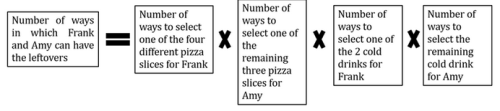Step 3: Determine the number of ways of doing each task

• Task 1 -Select one of the four different pizza slices for Frank

Number of ways of selecting 1 item from four different items = 4C1 = 4

Thus, there are 4 ways to do Task 1.

• Task 2 -Select one of the remaining three pizza slices for Amy

Number of ways of selecting 1 item from 3 different items = 3C1 = 3

Thus, there are 3 ways to do Task 2.

• Task 3 -Select one of the 2 cold drinks for Frank

Number of ways of selecting 1 item from 2 different items = 2C1 = 2

Thus, there are 2 ways to do Task 3.

• Task 4 -  Select the remaining cold drink for Amy

Number of ways of selecting 1 item from 1 item = 1

Thus, there is only 1 way to do Task 4.

Step 4: Calculate the final answer

(Number of ways in which Frank and Amy can have the leftovers)

= 4*3*2*1

= 24

So, there are 24 different ways in which Frank and Amy can eat the leftovers from the pizza party. .

QUESTION: 16

How many different 3-letter words can be formed from the letters L, M, N, O, P, and Q without repeating any letter? It is not necessary for the words to have meaning per the English dictionary.

Solution:

Understand the objective

• Find the different 3 letter word that can be formed using the letters  L, M, N, O, P and Q.
• (For example, words like LMN, MNP, LNM, PNQ etc.)

• Note that repetition of these letters is not allowed while framing a unique 3-letter word.
• (For example, numbers like PPQ, LLL, OPO etc. are not allowed)

• As we are arranging letters here to form unique 3-letter words this is clearly a permutation problem.

• We can either use Filling Space method or Permutation Formula to solve this problem.

Method 1: Filling Space method

Write the objective equation enlisting all tasks

There is a total of 6 letters: L, M, N, O, P and Q

To accomplish the objective of forming 3-letter words, three task needs to be done:

·       Task1: Selecting a letter for the first space

o   This task can be achieved in 6 ways.

(We can choose any of the six letters)

·       Task2: Selecting a number for the second space

o   This task can be achieved in 5 ways.

(We can choose among the remaining 5 letters since we already selected one for the first space)

·       Task3: Selecting a number for third space

o   This task can be achieved in 4 ways.

(We can choose among the remaining 4 letters since we already selected two one for the first space and other for the second space)

All these three tasks must be done to form three letter word.

Therefore,

• The objective of forming unique 3-letter word can be found by multiplying the number of ways in which each task is achieved.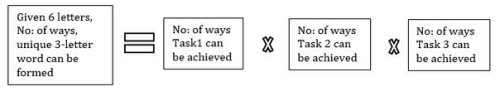Therefore,

·       No: of ways by which unique 3-letter words can be formed = 6 x 5 x 4 = 120 ways.

Method 2: Permutation Formula

We can also use the permutation formula (nPr) to find our answer.

Out of the given six letters (L, M, N, O, P, Q) we have to arrange three letters so as to make a unique 3-letter word.

Therefore,

• n = 6 and r = 3

Hence, the number of ways by which unique 3-digit numbers can be formed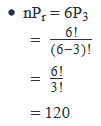QUESTION: 17

In how many ways can three different letters be selected from the letters of the word ABSOLUTE?

Solution:

Step 1: Understand the objective

The objective of the question is to find the number of ways in which three different letters can be SELECTED from a set of 8 different letters: A, B, S, O, L, U, T and E

Note that only selection is to be considered here. We don’t need to arrange these letters since the question did not ask us to make words.

Step 2: Write the objective equation enlisting all tasks

This is a Selection question. Order doesn’t matter here. So we will solve it by using the nCr formula.

In this case, only one task needs to be completed to accomplish the objective: Select 3 letters out of the given set of 8 different letters.

So, the objective equation becomes:

(Number of ways of selecting 3 letters out of 8 different letters) = 8C3

Step 3: Determine the number of ways of doing each task

By using the formula: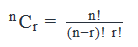We get,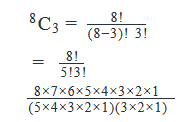Upon simplifying this expression we get

8C3=56

Step 4: Calculate the final answer

By putting this value in the objective equation, we get:

(Number of ways of selecting 3 letters out of 8 different letters) = 56

QUESTION: 18

The captain of a high school basketball team wants to assign unique three-digit number to all the team players. How many such unique numbers are possible if he decides to use only the even digits: 2, 4, 6 and 8 without repetition?

Solution:

Understand the objective

• Find the number of unique 3-digit numbers that can be formed using the numbers 2, 4, 6 and 8.
• (For example, numbers like 246, 468, 824 etc.)

• Note that repetition of these digits is not allowed while framing a unique 3-digit number.
• (For example, numbers like 226, 444, 884 etc. are not allowed)

• As we are arranging numbers here to form unique 3-digit numbers this is clearly a permutation problem.

• We can either use Filling Space method or Permutation Formula to solve this problem.

Method 1: Filling Space method

Write the objective equation enlisting all tasks

There is a total of four numbers: 2, 4, 6 and 8. To accomplish the objective of forming 3-digit numbers, three task needs to be done:

• Task1: Selecting a number for units’ digit
• This task can be achieved in 4 ways. (We can choose any of the four numbers)

• Task2: Selecting a number for tens digit.
• This task can be achieved in 3 ways.(We can choose among the remaining 3 numbers since we already selected one for the units’ digit)

• Task3: Selecting a number for hundreds digit.
• This task can be achieved in 2 ways. (We can choose among the remaining 2 numbers since we already selected two one for the units’ digit and other for the tens digit)

• All these three tasks must be done to form three-digit number.

Therefore,

The objective of forming unique 3-digit numbers can be found by multiplying the number of ways in which each task is achieved.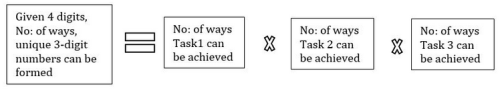Therefore,

• No: of ways by which unique 3-digit numbers can be formed = 4 x 3 x 2 = 24ways.

Method 2: Permutation Formula

We can also use the permutation formula (nPr) to find our answer.

Out of the given four numbers (2,4,6,8) we have to arrange three numbers so as to make a unique 3-digit number.

Therefore,

• n = 4 and r = 3

Hence, the number of ways by which unique 3-digit numbers can be formed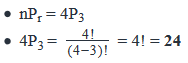QUESTION: 19

The captain of a high school basketball team wants to assign unique three-digit number to all the team players. How many such unique numbers are possible if he decides to use only the even digits: 0, 2, 4 and 6 without repetition?

Solution:

Understand the objective

• Find the number of unique 3-digit numbers that can be formed using the numbers 0, 2, 4 and 6.
• (For example, numbers like 206, 460, 624 etc.)

• Remember, we cannot use ‘0’ in the hundreds digit as we are asked to form three-digit numbers.
• (For example, 024, 046 are not three-digit numbers and hence not valid choices in this problem)

• Also, note that repetition of these digits is not allowed while framing a unique 3-digit number.
• (For example, numbers like 226, 444, 200, etc. are not allowed)

• As we are arranging numbers here to form unique 3-digit numbers this is clearly a permutation problem.
•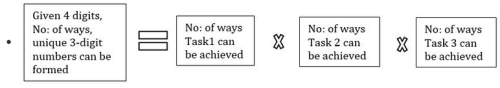• We can use Filling Space method or Permutation Formula to solve this problem.

Method 1: Filling Space method

Write the objective equation enlisting all tasks

There is a total of four numbers: 0, 2, 4 and 6

Similar to the previous problem, to accomplish the objective of forming 3-digit numbers, three task needs to be done:

Since we have a restriction of not using ‘0’ in the hundreds digit, we should first deal with that restriction.

Therefore,

·       Task1: Selecting a number for hundreds digit

o   This task can be achieved in 3 ways.

(We can choose from any 3 numbers: 2, 4 or 6. As we need a 3-digit number we cannot use ‘0’ at this place)

·       Task2: Selecting a number for tens digit

o   This task can be achieved in 3 ways.

(We can choose among the remaining 3 numbers since we already selected one for the hundreds digit)

·       Task3: Selecting a number for units’ digit

o   This task can be achieved in 2 ways.

(We can choose among the remaining 2 numbers since we already selected two: one for the hundreds digit and other for the tens digit)

·       All these three tasks must be done to form three-digit number.

Therefore,

·       The objective of forming unique 3-digit numbers can be found by multiplying the number of ways in which each task is achieved.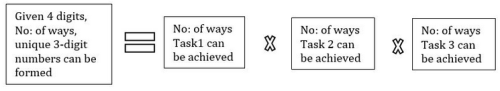Therefore,

• No: of ways by which unique 3-digit numbers can be formed = 3 x 3 x 2 = 18 ways.

Method 2: Permutation Formula

We can also use the permutation formula (nPr) to find our answer.

Out of the given four numbers (0, 2,4,6) we have to arrange three numbers so as to make a unique 3-digit number.

We also know that we cannot use 0 as hundreds digit.

For the time being, let us consider that ‘0’ can be the hundreds digit.

If we also include ‘0’ as hundreds digit, then we are simply arranging three numbers from a list of four numbers.

• The number of possibilities in that case would be 4P3 = 24

Now we know that among these 24 unique numbers, some numbers are those with ‘0’ as the hundreds digit. So, we need to subtract those.

If ‘0’ is fixed as the hundreds digit, then for the tens and units digit (2 places) we have the option to choose from remaining 3 numbers (2,4,6).

• This can be done in 3P2 ways = 6ways

• That is, out of the 24 possibilities, 6 are those numbers with ‘0’ as the hundreds digit.

Therefore,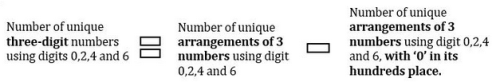Therefore,

• Number of unique three-digit numbers using digits 0,2,4 and 6 = 24 – 6 = 18

QUESTION: 20

In how many ways can three different letters be selected from the letters of the word THREE?

Solution:

Step 1: Understand the objective

The objective of the question is to find the number of ways in which three different letters can be SELECTED from a set of 4 letters: T, H, R and E.

(Please note that the word THREE has 5 alphabets, but of these, E occurs twice. So, we get only 4 different alphabets from the word THREE: T, H, R and E)

Step 2: Write the objective equation enlisting all tasks

This is a Selection question. Order doesn’t matter here. So we will solve it by using the nCr formula.

In this case, only one task needs to be completed to accomplish the objective: Select 3 letters out of the given set of 4 different letters.

So, the objective equation becomes:

(Number of ways of selecting 3 letters out of 4 different letters) = 4C3

Step 3: Determine the number of ways of doing each task

By using the formula: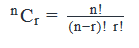We get: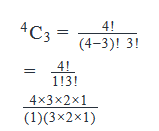Upon simplifying this expression, you get

4C3=4

Step 4: Calculate the final answer

By putting this value in the objective equation, we get:

(Number of ways of selecting 3 letters out of 4 different letters) = 4